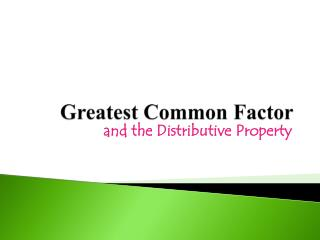Download PresentationGreatest Common Factor

# Greatest Common Factor - PowerPoint PPT PresentationDownload Presentation## Greatest Common Factor

- - - - - - - - - - - - - - - - - - - - - - - - - - - E N D - - - - - - - - - - - - - - - - - - - - - - - - - - -
##### Presentation Transcript

1. Greatest Common Factor and the Distributive Property

2. Greatest Common Factor • You previously learned about the distributive property, but in case you have forgotten about it… • Given two terms, the first being a number and the second the sum of two numbers, the product of the two terms is the same as the sum when multiplying each of the addends by the first term. • Example: 5(4 + 3) = • You can use the distributive property in combination with the concept of GCF to re-write multiplication expressions. 5 •4 + 5 •3

3. How To… Example 1: Express the sum of 15 and 25 as the product of the GCF and the sum of their respective factors. First, find the GCF of 15 and 25: _____ Next, write the GCF as the term outside the parenthesis:__ ( __ + __ ) Finally, write the factor that when multiplied by the term outside the parenthesis will give you the original numbers. __ ( __ + __ ) 5 5 4 5 3

4. How To… Example 2: Express the sum of 36 and 66 as the product of the GCF and the sum of their respective factors. First, find the GCF of 36 and 66: _____ Next, write the GCF as the term outside the parenthesis:__ ( __ + __ ) Finally, write the factor that when multiplied by the term outside the parenthesis will give you the original numbers. __ ( __ + __ ) 6 6 11 6 6

5. More Examples Example 1: Express the sum of 16 and 64 as the product of the GCF and the sum of their respective factors. First, find the GCF of 36 and 66: _____  Next, write the GCF as the term outside the parenthesis:__ ( __ + __ ) Finally, write the factor that when multiplied by the term outside the parenthesis will give you the original numbers. __ ( __ + __ ) 16 16 4 16 1

6. More Examples Example 1: Express the sum of 32 and 96 as the product of the GCF and the sum of their respective factors. First, find the GCF of 32 and 96: _____  Next, write the GCF as the term outside the parenthesis:__ ( __ + __ ) Finally, write the factor that when multiplied by the term outside the parenthesis will give you the original numbers. __ ( __ + __ ) 32 32 3 32 1